# Probability

(diff) ← Older revision | Latest revision (diff) | Newer revision → (diff)

mathematical

A numerical characteristic expressing the degree to which some given event is likely to occur under certain given conditions which may recur an unlimited number of times. As a category of scientific knowledge, the concept of "probability" describes a special type of connection between the phenomena which are typical of mass processes. The concept of "probability" forms the foundation of the special system of theorems that constitute probability theory and mathematical statistics.

The numerical value of a probability may sometimes be obtained from its "classical" definition: The probability is equal to the quotient of the number of cases "favouring" a certain event to the total number of "equally possible" cases. For instance, if, out of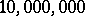government bonds, one of which carries a maximum prize,were bought in a certain city, the probability of an inhabitant of that city winning the prize is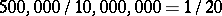.

In other, more complicated cases, a statistical approach is needed to determine the numerical value of a probability. For instance, if a marksman has hit the target in 39 out of 100 shots, it may be thought that the probability of his hitting the target under these conditions is approximately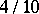. Both the classically and statistically determined probabilities may be used to compute new probabilities, in accordance with the laws of probability theory. For instance, if the marksman can hit the target with a probability of, the probability of his hitting the target at least once in four shots will be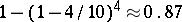. This conclusion may be statistically verified: If trials aimed at hitting the target at least once out of four shots are repeated a sufficient number of times, they will be successful in aboutof the cases (provided there is no significant change in the skill of the marksman during that time).

Mathematical probability is an expression of the qualitatively specific relation between random and non-random events. In developing probability theory, those properties of a probability which at the present stage of development of the theory are necessary to ensure further advance are formulated as axioms. However, neither these axioms nor the classical approach to probability nor the statistical approach fully explains the real meaning of the concept of "probability" ; they are merely approximations to a more and more complete description. Certainly not every event whose occurrence is not uniquely determined under given conditions has a definite probability under these conditions. The assumption that a definite probability (i.e. a completely defined fraction of the number of occurrences of an event if the conditions are repeated a large number of times) in fact exists for a given event under given conditions is a hypothesis which must be verified or justified in each individual case. For instance, it is meaningful to speak about the probability of hitting a target of a certain size, from a given distance, with a given type of rifle by a marksman picked at random from a given military formation. However, it would be meaningless to speak of the probability of hitting a target if nothing is known of the firing conditions.

As regards the connection between probability and frequency, one must bear the following in mind: If the number of repetitionsof the given conditions is finite, the fraction of the number of occurrences of the given event, i.e. the so-called frequency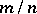, usually differs but little from the probability. The larger the number of repetitions, the rarer become any significant deviations of the frequencyfrom the probability. To illustrate this point, consider the case of tossing a coin, where the probabilities of "heads" and "tails" are both equal to. If the coin is tossed 10 times (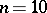), it is not very probable that one will obtain 10 "heads" or 10 "tails" . Neither is there sufficient reason to maintain that "heads" will come out exactly 5 times; even if one predicts 4 or 5 or 6 "heads" , one runs a substantial risk of being wrong. However, for 100 tosses it is possible to tell in advance, without incurring any significant risk, that the number of "heads" will be between 40 and 60 (cf. Law of large numbers).

The mathematical probability may serve as an estimate of the probability of an event in the ordinary, everyday-life sense, i.e. it may render more precise "problematic" statements usually expressed by the words "possibly" , "probably" , "very probably" , etc. As regards such estimates, it must be borne in mind that if they are applied to a statement which, by its very nature, can only be true or false, the estimate of its probability can only have a temporary or a subjective meaning, i.e. it merely reflects our attitude in the matter. For instance, a person without any information on the subject wishing to imagine the environmental outlook of Moscow on the 23rd March 1930, may say: "On that day, snow probably still covered the fields" . Actually, however, the snow on the fields near Moscow had vanished already on March 22. If one is made aware of this fact, the original statement — the problematic statement between inverted commas — must be modified. Nevertheless, that statement, though erroneous in this particular case, is based on a valid general rule: "During the first twenty-or-so days in March the fields around Moscow are usually covered with snow" . This rule reflects the objective characteristics of the climate around Moscow. Such rules may be expressed by indicating the probability level of the event in question under certain general conditions, which can be realized an unlimited number of times. Such estimates do have an objective meaning. Accordingly, the calculation of mathematical probability in order to arrive at a degree of reliability of certain statements concerning individual events is no longer a mere expression of the subjective belief that the event will or will not take place. Such an idealistic, subjective understanding of the sense of mathematical probability is erroneous. If pursued to its logical conclusion, it would result in the absurd claim that valid conclusions about the world around us can be arrived at in complete ignorance, by merely analyzing subjective, more or less reliable opinions.

The use of calculated probabilities to estimate the situation in individual cases necessarily gives rise to the question as to what probabilities may be ignored in practice. This problem may be solved in different ways, depending on the necessity for a rapid transition from the accumulation of reliable data to their actual application. For instance, if under given circumstances a military problem can be solved by a given number of shots with a probability of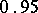(i.e. if the probability of the given number of projectiles not being sufficient is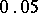), it is usual to conduct the military mission on the assumption that the number of shots is sufficient. Under the more peaceful conditions of scientific research it is usual to neglect only probabilities as small as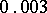(this is connected with the so-called three-sigma-rule), and on certain occasions the probability of an outcome, for it to be termed "certain" , must be even closer to one. In mathematical statistics, the probability which one decides to ignore in a given study is said to be the significance level. In statistics, the recommended significance level varies fromfor preliminary orientation experiments to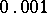for important ultimate conclusions, but the attainable reliability of probability conclusions is often much higher. Thus, the principal conclusions of statistical physics are based on the neglect of probabilities of an order less than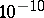.

The mathematical models employed in probability theory are based on three concepts: A spaceof so-called elementary events, a class of subsets of(events) and a probability distribution — a set function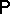defined on this class. The value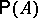ofat an eventis then called the probability of.

How to Cite This Entry:
Probability. Encyclopedia of Mathematics. URL: http://encyclopediaofmath.org/index.php?title=Probability&oldid=12113
This article was adapted from an original article by A.N. Kolmogorov (originator), which appeared in Encyclopedia of Mathematics - ISBN 1402006098. See original article